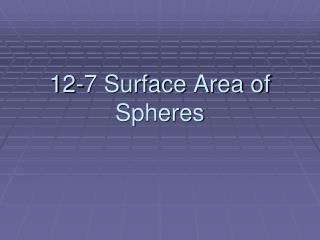DownloadDownload Presentation12-7 Surface Area of Spheres

# 12-7 Surface Area of Spheres

Télécharger la présentation## 12-7 Surface Area of Spheres

- - - - - - - - - - - - - - - - - - - - - - - - - - - E N D - - - - - - - - - - - - - - - - - - - - - - - - - - -
##### Presentation Transcript

1. 12-7 Surface Area of Spheres

2. Objective • Recognize and define basic properties of spheres • Find surface area of spheres

3. Spheres • Sphere – In space, infinitely many points that are a given distance from a central point. • Great Circle – the circle made when a plane intersects a sphere where it contains the center. A great circle has the same center and radii of the sphere it is within. • Hemisphere – one of two halves of a sphere. Each great circle in a sphere separates it into two hemispheres. Great Circle

4. Example 1 In the figure, O is the center of the sphere and plane R intersects the sphere In circle A. If AO is 3 cm and OB is 10 cm, find AB B R A o

5. 2 2 2 • The radius of circle A is segment AB, B is a point on circle A and on sphere O. Use Pythagorean Theorem for the right triangle ABO to solve for AB • OB = AB + AO • 10 = AB + 3 • 100 = AB + 9 • 91 = AB • 9.5 AB Pythagorean Theorem 2 2 2 OB = 1=, AO = 3 2 Simplify 2 Subtract 9 from each side ~ Use a calculator ~ AB is approximately 9.5 centimeters

6. Surface Area of Spheres • If a sphere has a surface area of T units and a radius of r units then T = 4 or 4 times the area of the sphere’s great circle. r 2 2 (4)( )(r) = surface area

7. Example 2 Find the surface area of the sphere given the area of the great circle 2 A 314.2 in ~ ~

8. r 2 Surface area of a sphere. • From the formula, we know that the area of the sphere is 4 times the area of its great circle. • T = 4 • T = 4(314.2) • T = 1256.8 in • The surface area is about 1256.8 in Surface area of GS is approx. 314.2 2 Multiply 2

9. Example 3 Find the surface area of a baseball with a circumference of 9 inches To determine how much leather is needed to cover the ball. 9 inches

10. Circumference of a circle • We know that the circumference is 9 so we can use that to find the area of the great circle • 9 = D • D 1.4 • T = 4 r • T = 4 (1.4) • T 25.8 ~ Use a calculator ~ 2 Surface area of a sphere 2 r = 1.4 ~ Use a calculator ~ The surface area is approx. 25.8 sq. inches

11. Assignment • Pg 673 • 10-15, 17-31